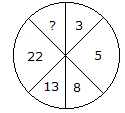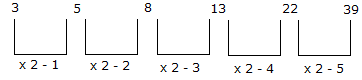# Verbal Reasoning - Character Puzzles - Discussion

### Discussion :: Character Puzzles - Character Puzzles 1 (Q.No.13)

13.

Which one will replace the question mark ?[A]. 45 [B]. 29 [C]. 39 [D]. 37

Explanation:Karps said: (Dec 31, 2011) (3(4)5) in this in between there is 1 number. (5(6,7)8) in this in between there is 2 number 2^1=2. (8(9,10,11,12)13) in this in between there is 4 number is 2^2=4. (13(14,15,16,17,18,19,20,21)22)in this in between there is 8 number 2^3=8. (22(23,24,25,26,27,28,29,30,31,32,33,34,35,36,37,38)39) in this in between there is 16 number 2^4=16.

 Mohanapriya said: (Jun 2, 2012) Its simple 3+3=6 6-1=5 5+5=10 10-2=8 8+8=16 16-3=13 13+13=26 26-4=22 Therefore 22+22=44 44-5=39

 Pranesh Kumar said: (Jun 10, 2012) Thank you !mohana priya.

 Vikram said: (May 3, 2016) Thank you @Mohanapriya.

 Illeana said: (May 31, 2016) Very interesting problem and good explanations.

 Vasanth said: (Aug 13, 2016) You are genius @Mohanapriya.

 Baye said: (Nov 1, 2016) Thank you for explaining the solution.

 Vasanthi said: (Mar 15, 2017) But I got the answer is 35. Can anyone help me?

 Vasanthi said: (Mar 15, 2017) 3 + 5 = 8 5 + 8 = 13 8 + 13 = 22 13 + 22 = 35 The answer is 35.

 Ratnakar Majhi said: (Mar 19, 2017) Can anyone explain the solution to understand the answer?

 Venu Reddy said: (Mar 24, 2017) Yes, right @Vasanthi.

 Ravi said: (Mar 27, 2017) Very nice and thank you very much.

 B.Balaji Naik said: (Apr 9, 2017) @Vasanthi. Answer is wrong because 8+13 is not equal to 22. You are correct @Mohanapriya.

 Dharmik said: (Apr 14, 2017) Your are right @Mohanapriya.

 Sourav Mohanta said: (Apr 22, 2017) Thank you all for the given explanation.

 Hisafaaz said: (Apr 30, 2017) Thanks for the intelligent answer @Mohanapriya.

 Devvrat said: (May 4, 2017) Thanks for giving the easy solution.

 Muhammad Zeeshan said: (May 14, 2017) Thank you @Mohanapriya.

 Kiruba Prakash said: (May 15, 2017) 3+3=6 6-1 = 5. 5+5=10 10-2 = 8. 8+8=16 16-3 = 13. 13+13=26 26-4 = 22. Then, 22+22 = 44 44-5 = 39.

 Prasanth said: (May 18, 2017) Mohana priya, and kirubaaa nice explain.

 Karthik said: (May 20, 2017) Thanks for the giving explanation.

 Uday Shankar said: (May 20, 2017) Well said, Thanks @Mohanapriya & @Kiruba.

 Kuldeep Saini said: (May 22, 2017) 0 + 3 = 3. 3 + 3 = 6 - 1, 5. 5 + 5 = 10 - 2.8. 8 + 8 = 16 - 3 = 13. 13 + 13 = 26 - 4, 22. 22 + 22 = 44 - 5, 39.

 Kanishkha said: (May 23, 2017) Thanks for your explanation @Kuldeep.

 Shivani Kukreja said: (May 25, 2017) Nic explanation @Mohanapriya.

 Usha said: (Jun 4, 2017) Can't understand it, please explain it in clear.

 Vijaya said: (Jun 8, 2017) 3 * 2 = 6 - 1 = 5, 5 * 2 = 10 - 2 = 8, 8 * 2 = 16 - 3 = 13, 13 * 2 = 26 - 4 = 22, 22 * 2 = 44 - 5 = 39.

 Dibyaranajn said: (Jun 8, 2017) Nice explanation, Thank you @Mohanapriya.

 Seetha said: (Jun 11, 2017) Nice explanation, thank you all.

 Malaya Tandi said: (Jun 22, 2017) Agree, and thanks for your solution @Kuldeep.

 Poojaawasthi said: (Jun 23, 2017) Nice explanation, thanks.

 Rajashekar said: (Jul 14, 2017) 3 - 5 - 8 - 13 - 22 - ? Difference. : 2---3---5---9---(17). Internal Diff:. 1 - 2 - 4 - 8 (Double Previous), ? = 22+17 = 39.

 R@Ju Mhr said: (Jul 23, 2017) @Mohanapriya. Nice explanation. Thanks.

 Bishen said: (Mar 14, 2019) @Vasanthi and @Venu Reddy. 35 is wrong. Actually, 8+13=21.

 Tarani said: (Dec 22, 2019) I think correct answer is 39. Actually 8+13 is not 22 it's 21.

 Rakshit Sharma said: (Apr 26, 2020) Thank you all for explaining this problem.